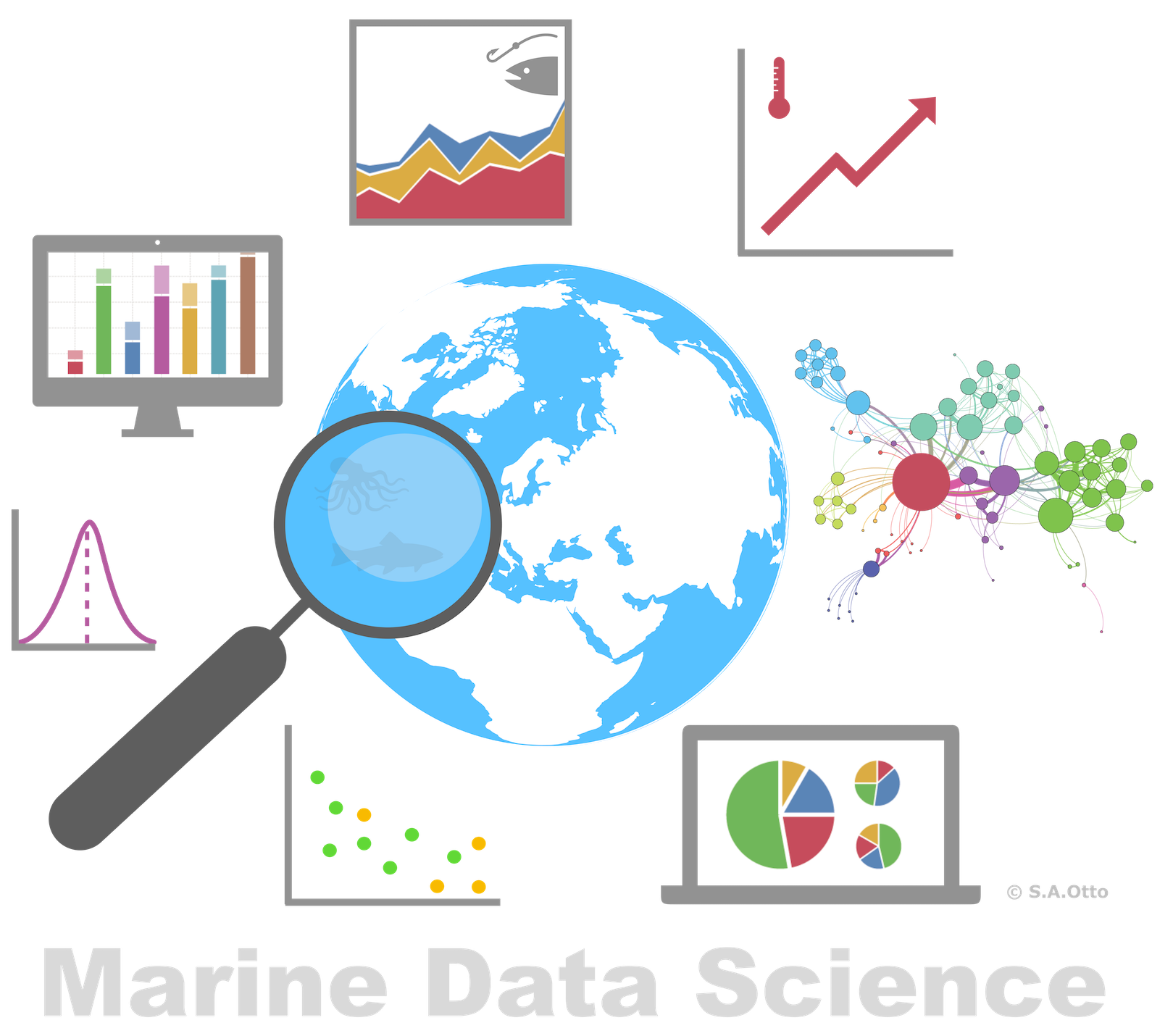Data Analysis with R

18 - Iteration 2 (purrr and the map family)

Postdoctoral Researcher

Functional programming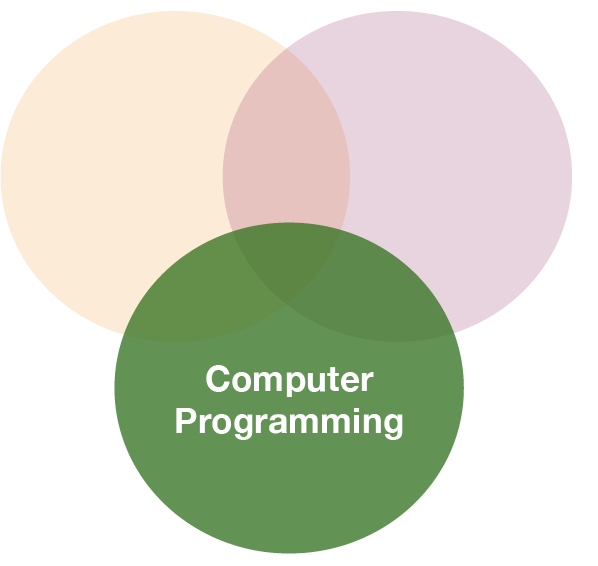Loops

• for loops are not as important in R as they are in other languages because R is a functional programming language.
• It is possible to wrap up for loops in a function, and call that function instead of using the for loop directly.

Consider (again) this simple data frame: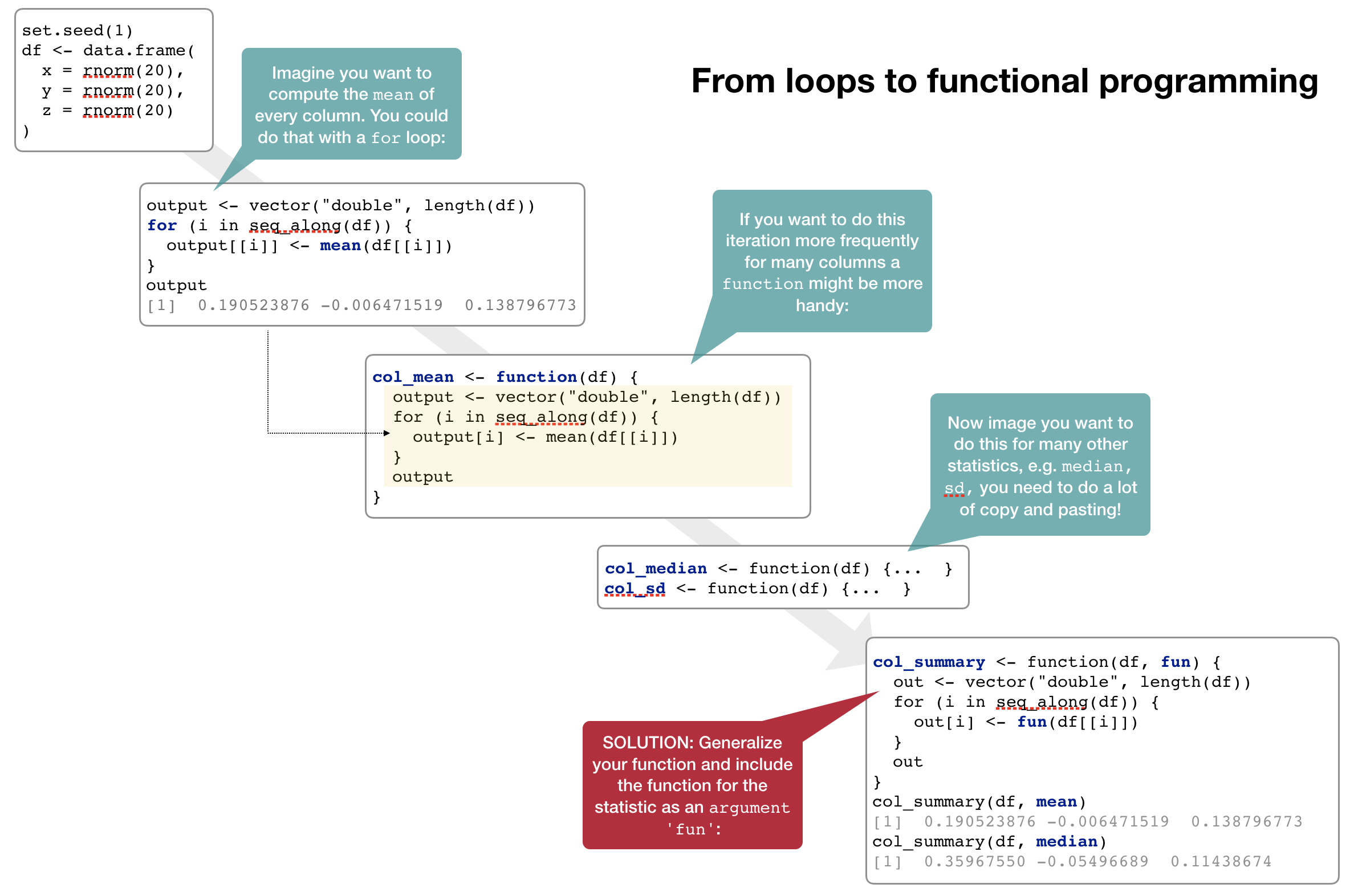Functional programming with purrr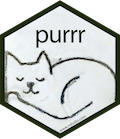• As you have seen, passing a function to another function is extremely handy, reduces potential bugs (much less code and copy and pasting), and makes it easy to generalise
• The apply family of functions in base R (apply(), lapply(), sapply(), vapply(), tapply(), mapply()) does exactly that: Thes functions act on an input list, vector, dataframe, matrix or array, and apply a named function with one or several optional arguments.
• The map family of functions provided by the tidyverse packages purrr operates similar but can be faster (all functions written in C++), is more consistent, well integrated in the tidyverse concept and easier to learn.
• purrr provides in addition many more useful functions for handling lists; to have an overview of available functions see the cheatsheet

The most basic function: map()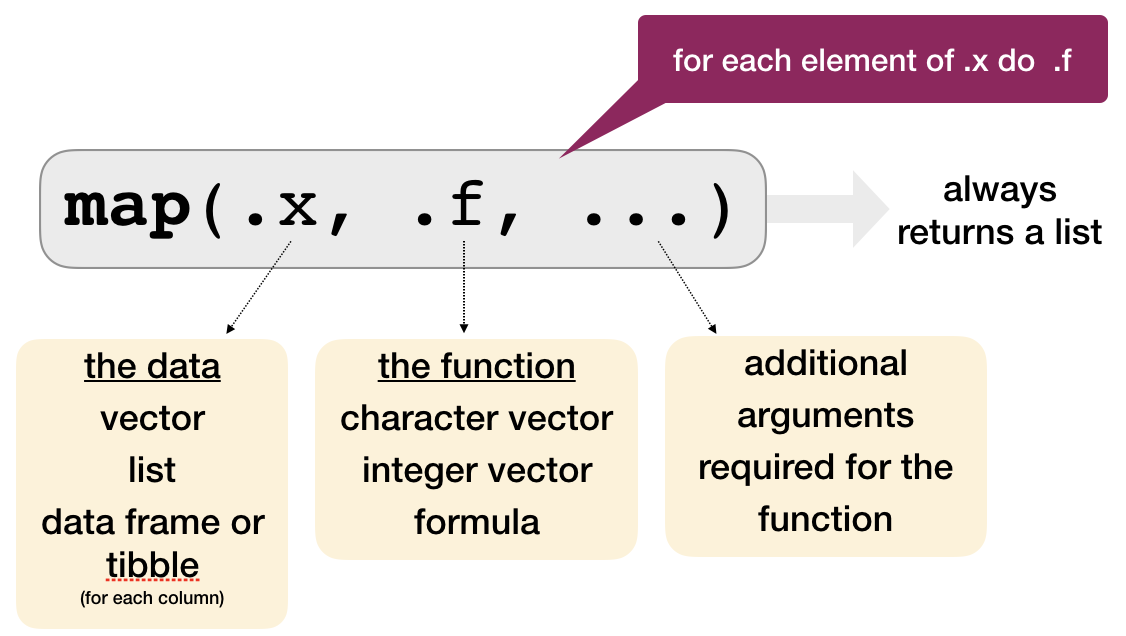The most basic function: map()

Using our previous example:

set.seed(1)
df <- data.frame(
x = rnorm(20),
y = rnorm(20),
z = rnorm(20)
)

map() always preserves the list names.

map(df, mean)
## $x ##  0.1905239 ## ##$y
##  -0.006471519
##
## $z ##  0.1387968 # You can also use the pipe operator df %>% map(median) The ... argument Here you specify all other arguments which can be specified in the function used: map(df, quantile, probs = c(0,0.5,1.0) ) ##$x
##         0%        50%       100%
## -2.2146999  0.3596755  1.5952808
##
## $y ## 0% 50% 100% ## -1.98935170 -0.05496689 1.35867955 ## ##$z
##         0%        50%       100%
## -1.1293631  0.1143867  1.9803999

Other types of output than a list

• map_lgl() → returns a logical vector
• map_int() → returns an integer vector
• map_dbl() → returns a double vector
• map_chr() → returns a character vector

The length of the returned vector and .x are always the same!

To get the means of x, y and z as vector replace map() with the appropriate function:

map_dbl(df, mean)
##            x            y            z
##  0.190523876 -0.006471519  0.138796773

Note: :

map_dbl() returns a named vector based on the original list names!

Other types of output than a list

You can always generate a vector of a more general data type but not the opposite

map_int(df, mean)
## Error: Can't coerce element 1 from a double to a integer
map_chr(df, mean)
##           x           y           z
##  "0.190524" "-0.006472"  "0.138797"

Task: Explore the data

Load the following R datafile, which contains the list groundsharks:

ls()
##  "groundsharks"

This list contains data for 284 groundshark species (Carcharhiniformes, the largest order of sharks) downloaded from fishbase. The list has a hierarchical structure with one list per species containing individual sublists for each information.

Task: Explore the data

Answer the following questions

1. How many elements are in groundsharks?
2. What is the first species listed in groundsharks? What information is given for this species?
3. What is the difference between groundsharks and groundsharks[]?

(Answers are on the next slide)

length(groundsharks[])
##  99
names(groundsharks[])
##   "sciname"              "Genus"                "Species"
##   "SpeciesRefNo"         "Author"               "FBname"
##   "PicPreferredName"     "PicPreferredNameM"    "PicPreferredNameF"
##  "PicPreferredNameJ"    "FamCode"              "Subfamily"
##  "GenCode"              "SubGenCode"           "BodyShapeI"
##  "Source"               "AuthorRef"            "Remark"
##  "TaxIssue"             "Fresh"                "Brack"
##  "Saltwater"            "DemersPelag"          "AnaCat"
##  "MigratRef"            "DepthRangeShallow"    "DepthRangeDeep"
##  "DepthRangeRef"        "DepthRangeComShallow" "DepthRangeComDeep"
##  "DepthComRef"          "LongevityWild"        "LongevityWildRef"
##  "LongevityCaptive"     "LongevityCapRef"      "Vulnerability"
##  "Length"               "LTypeMaxM"            "LengthFemale"
##  "LTypeMaxF"            "MaxLengthRef"         "CommonLength"
##  "LTypeComM"            "CommonLengthF"        "LTypeComF"
##  "CommonLengthRef"      "Weight"               "WeightFemale"
##  "MaxWeightRef"         "Pic"                  "PictureFemale"
##  "LarvaPic"             "EggPic"               "ImportanceRef"
##  "Importance"           "PriceCateg"           "PriceReliability"
##  "Remarks7"             "LandingStatistics"    "Landings"
##  "MainCatchingMethod"   "II"                   "MSeines"
##  "MGillnets"            "MCastnets"            "MTraps"
##  "MSpears"              "MTrawls"              "MDredges"
##  "MLiftnets"            "MHooksLines"          "MOther"
##  "UsedforAquaculture"   "LifeCycle"            "AquacultureRef"
##  "UsedasBait"           "BaitRef"              "Aquarium"
##  "AquariumFishII"       "AquariumRef"          "GameFish"
##  "GameRef"              "Dangerous"            "DangerousRef"
##  "Electrogenic"         "ElectroRef"           "Complete"
##  "GoogleImage"          "Profile"              "PD50"
##  "Emblematic"           "Entered"              "DateEntered"
##  "Modified"             "DateModified"         "Expert"
##  "DateChecked"          "TS"                   "SpecCode"

Quiz 1: What is the longevity of each species in the wild?

(this information is stored in LongevityWild)

STRATEGY

1. Do it for one element
2. Turn it into a recipe
3. Use map() to do it for all elements

1. What is the longevity for the blacknose shark (the first species in the list)?

• Solve the problem for one element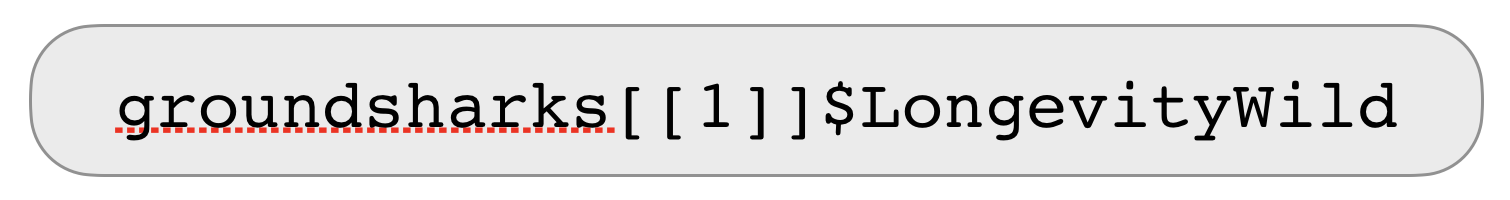2. Turn it into a receipe

• Make it a formula
• Use .x as a pronoun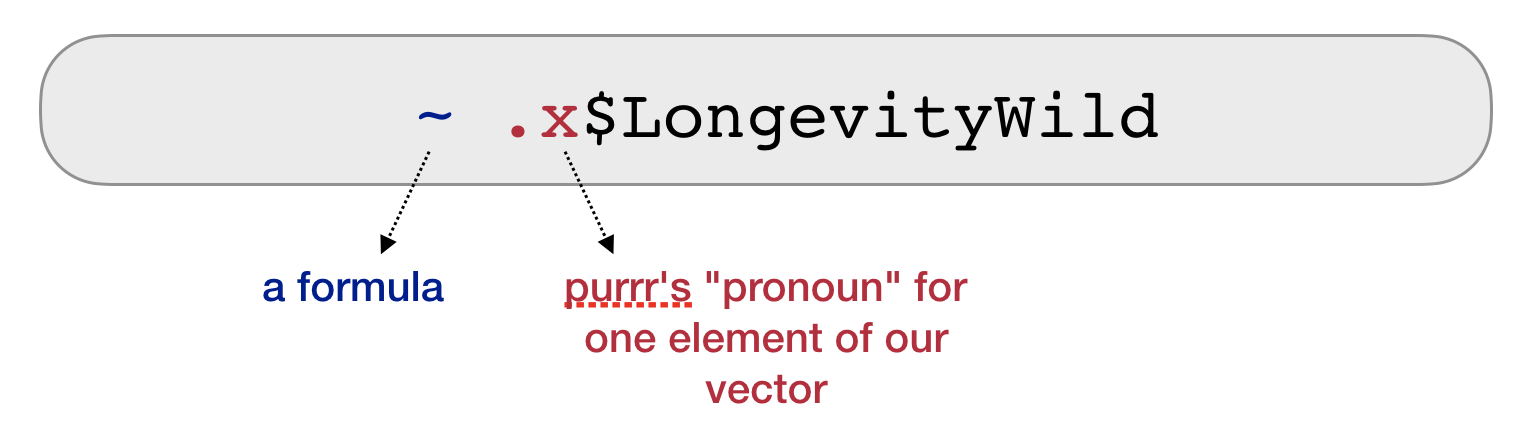3. Do it for all elements

• Your recipe is the second argument to map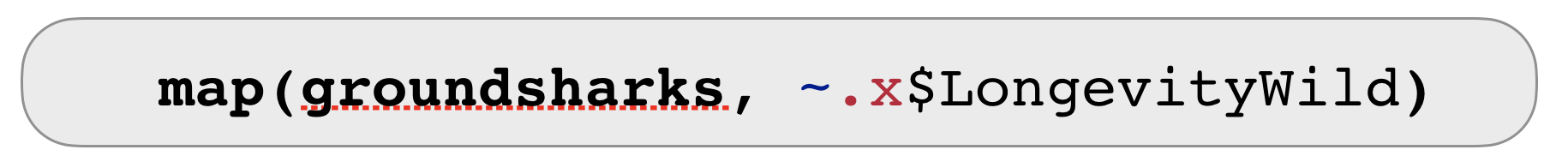Quiz 2: What is the mean longevity in the wild across all shark and ray species?

Solution: What is the mean longevity in the wild across all shark and ray species?

Applying a mean to a list is difficult ➔ use map_dbl() to get a vector returned:

map_dbl(groundsharks, ~ .x$LongevityWild) %>% mean(na.rm = TRUE) ##  20.125 Ways of specifying .f.f can be a formula map_int(groundsharks, ~ length(.x)) %>% head() ##  99 99 99 99 99 99 map_chr(groundsharks, ~ .x[["FBname"]]) %>% head() ##  "Blacknose shark" "Silvertip shark" "Bignose shark" ##  "Graceful shark" "Blacktail reef shark" "Pigeye shark" map_chr(groundsharks, ~ .x$FBname) %>% head()
##  "Blacknose shark"      "Silvertip shark"      "Bignose shark"
##  "Graceful shark"       "Blacktail reef shark" "Pigeye shark"

.f can be a string or integer

• For each element, extract the named or numbered element.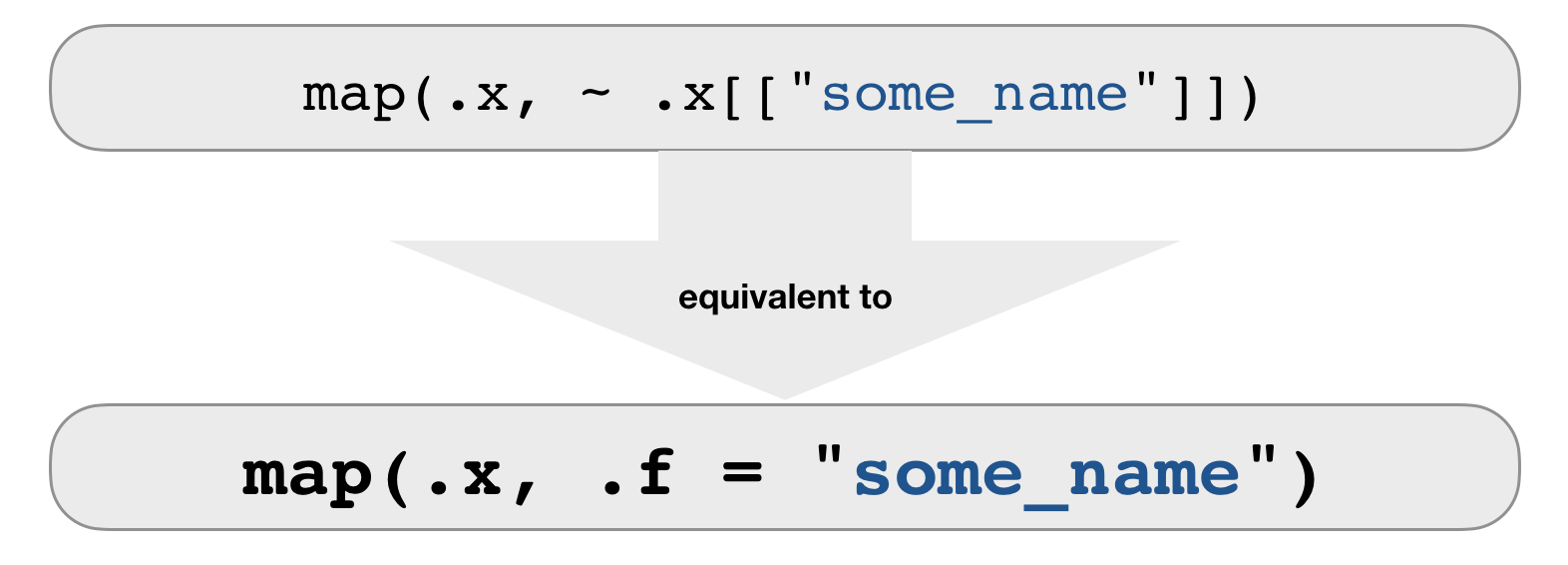map_chr(groundsharks, .f = "FBname")

# use an integer to select elements by position:
map(groundsharks, 97)

.f can be a function

• For each element, extract the named or numbered element.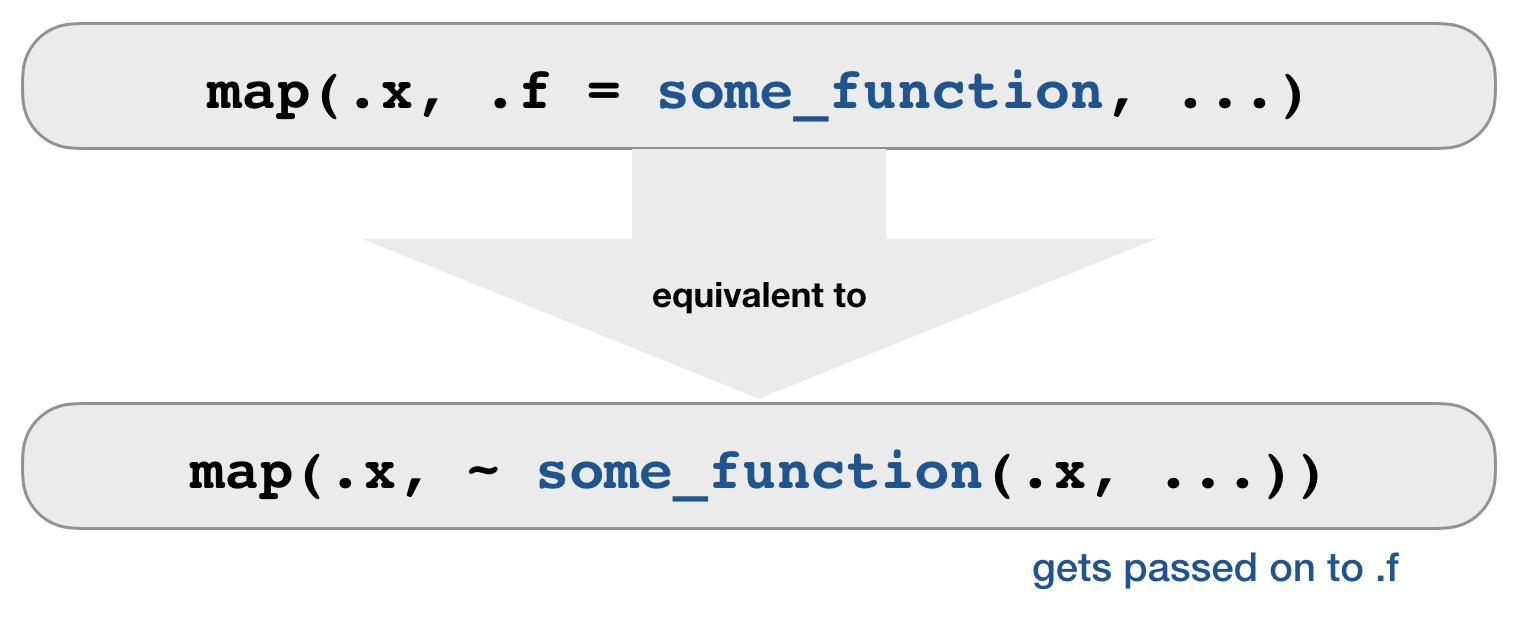map(.x = df, .f = mean, na.rm = TRUE)
map(.x = df, ~ mean(.x, na.rm = TRUE))

Combining map functions

How long is each species name?

# 1. Extract the scientific species name
char_species <- map(groundsharks, "sciname")
# 2. Get the length of the name (= number of characters)
map_int(char_species, str_length) %>% head()
##  22 27 20 29 26 24
# Piping both map functions
map(groundsharks, "sciname") %>% map_int(str_length) %>% head()
##  22 27 20 29 26 24
# Now in one go
map_int(groundsharks, ~ str_length(.x[["sciname"]])) %>% head()
##  22 27 20 29 26 24

set_names()

is a useful function for extracting information from sublists and using this to set the names of another list.

• Example: Get the corresponding scientific name to each length value:
# First extract the length values ...
map_dbl(groundsharks, .f = "Length") %>%
# ...and give it the names from sciname
set_names(map_chr(groundsharks, .f = "sciname")) %>% head()
##        Carcharhinus acronotus   Carcharhinus albimarginatus
##                           200                           300
##          Carcharhinus altimus Carcharhinus amblyrhynchoides
##                           300                           161
##    Carcharhinus amblyrhynchos      Carcharhinus amboinensis
##                           255                           280

Quiz 3: Extract more information from 'groundsharks'

1. Which species has the highest weight?
2. Which species has the lowest vulnerability score?
3. Which species swims deepest?
4. Which species do we know the least about (i.e. have the most NA entries)?

Solutions 1 + 2

# Species with the highest weight
map_dbl(groundsharks, .f = "Weight") %>%
set_names(map_chr(groundsharks, .f = "FBname")) %>%
sort() %>% tail(n = 1)
## Tiger shark
##      807400
# Species with the lowest vulnerability score
map_dbl(groundsharks, .f = "Vulnerability") %>%
set_names(map_chr(groundsharks, .f = "FBname")) %>%
sort() %>% head(n = 1)
## Pygmy ribbontail catshark
##                     12.55

Solutions 3 + 4

# Species that swims deepest
map_dbl(groundsharks, .f = "DepthRangeDeep") %>%
set_names(map_chr(groundsharks, .f = "FBname")) %>%
sort() %>% tail(n = 1)
## Silky shark
##        4000
# Species we know least about
map_int(groundsharks, ~ sum(is.na(.x))) %>%
set_names(map_chr(groundsharks, .f = "sciname")) %>%
sort() %>% tail(n = 1)
## Haploblepharus kistnasamyi
##                         60

Other iteration functionsMapping over 2 arguments: map2()• map2() applies a function to PAIRS of elements from two lists, vectors, etc.
• to get a vector returned: map2_lgl(), map2_int(), map2_dbl(), map2_chr()

Mapping over 2 arguments: map2()

map2(.x = c("a","b","c"), .y = c(1,2,3),  .f = rep)
## []
##  "a"
##
## []
##  "b" "b"
##
## []
##  "c" "c" "c"

Mapping over multiple arguments: pmap()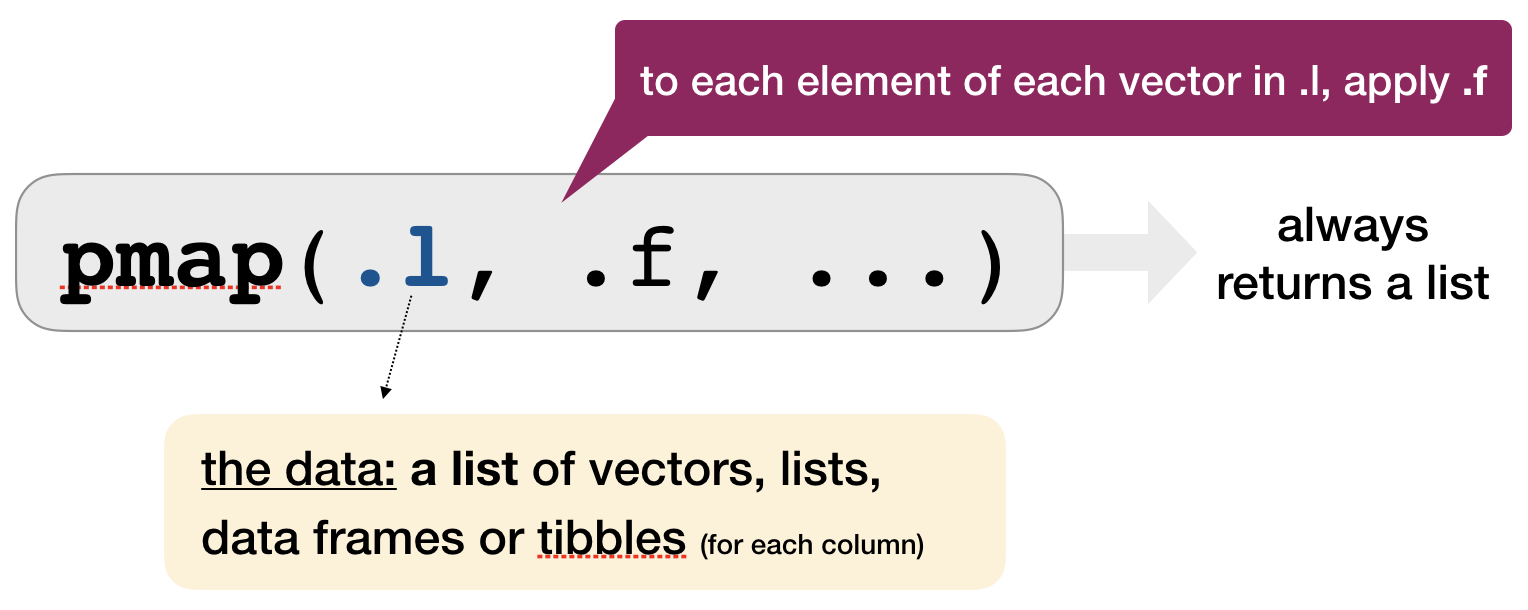• pmap() applies a function to GROUPS of elements FROM a LIST of lists, vectors, etc.
• NO corresponding pmap_lgl(), pmap_int(), etc.

Mapping over multiple arguments: pmap()

Example: sample from these 3 vectors 2, 10, or 5 times with or without replacement

arg_list <- list(x = list(a = 1:10, b = 1:5, c = 1:20), size = c(2, 10, 5),
repl = c(FALSE, TRUE, FALSE))
pmap(.l = arg_list, .f = sample)
## $a ##  10 5 ## ##$b
##   3 1 4 3 3 2 2 3 3 1
##
## $c ##  1 13 17 11 9 One more step in complexity: Invoking different functions with invoke_map() As well as varying the arguments to the function you might also vary the function itself. Example: Apply the mean, median and sd to a single vector or to a list: # Single vector invoke_map(.f = list(mean, median, sd), x = 1:10) ## [] ##  5.5 ## ## [] ##  5.5 ## ## [] ##  3.02765 # List params <- list(alist(x= 1:15), list(x=200:400), list(x=1:4)) invoke_map_dbl(.f = list(mean, median, sd), .x = params) ##  8.000000 300.000000 1.290994 Other mapping functions • invoke(), • lmap(), • imap(), • walk(), walk2(), pwalk() for side effects (returns input invisibly) Your turn... Lets go back to the exercise in the previous lecture on loops: Write a function to fit a linear model to each data.frame (column x vs column y) and plot the slopes as histogram. You can use multiple files ("ex_final_multifile_1.csv", "ex_final_multifile_2.csv", ...) or a single file ("ex_final_one_file.csv") to solve this.... Lets tackle this task with purrr: • import "dummyfile_1.csv" - "dummyfile_100.csv" so that you have one data list • apply the linear model to each dataframe using one of the map functions • extract the slopes and create a histogram Solution # 1. Import files <- str_c("data/functions/dummyfile_", 1:100, ".csv") data_list <- map(files, read_csv) # Lets add a column with the name of the file data_list <- map2(.x = data_list, .y = as.character(1:100), ~ mutate(.x, dataset = .y)) # 2. Apply linear models, extract slope and plot the histogram map(data_list, ~ lm(y ~ x, data = .x)) %>% map_dbl(~ coef(.x)) %>% hist(main = "Distributions of slopes")purrr and list-columnslist-columns • Data frames are a extremely handy data structure for data analysis: • they are more clearly structured than a list (similar to a matrix) • but they can contain different data types (which the matrix cannot) • being a hybrid between a list and matrix allows a very flexible usage, e.g. dataframes can be indexed like a list or a matrixs • We typically regard data frames a container for several atomic vectors of the same or different data type with a common length. • But data frames are even more flexible! They can contain all vector types, that includes list (recall: atomic vectors and lists represent together vectors!) • Such list in a data frame are called list-columns • You can even store individual ggplot objects in list-columns! list-columns • Tibbles are particularly good in handling and visualizing list-columns: ## # A tibble: 100 x 2 ## dataset data ## <chr> <list> ## 1 1 <tibble [100 × 2]> ## 2 2 <tibble [100 × 2]> ## # … with 98 more rows How to make a dataframe with list-columns • Use tidyr::nest() to create a nested dataframe in which individual tables are stored within the cells of a larger table. • Use a 2-step approach: first group the data, then create the nested data with one row per group level. # First convert data list into a tibble df <- data_list %>% bind_rows() # 2-step approach df_nested <- df %>% group_by(dataset) %>% nest()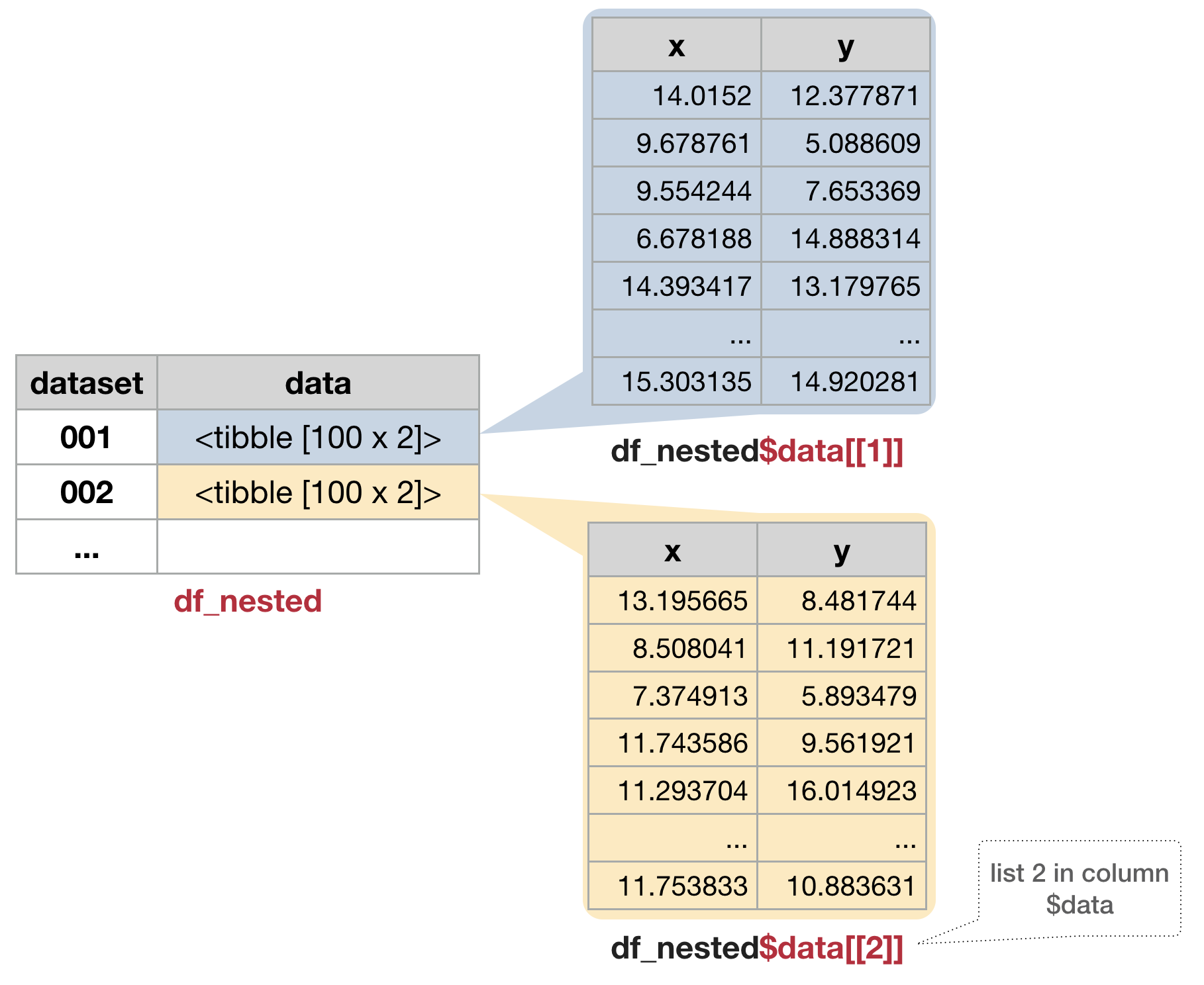Map data-frames to the modeling function • Nested data frames are useful when you want to preserve the relationships between observations and subsets of data. • You can manipulate many sub-tables at once with the purrr mapping functions and save results as list-column in the same dataset: model_nested <- df_nested %>% mutate(model = map(data, ~lm(y ~ x, data = .))) %>% print(n = 2) ## # A tibble: 100 x 3 ## dataset data model ## <chr> <list> <list> ## 1 1 <tibble [100 × 2]> <S3: lm> ## 2 2 <tibble [100 × 2]> <S3: lm> ## # … with 98 more rows Extract some summary statistics using piped operations stats_nested <- model_nested %>% mutate( alpha = map(model, coef) %>% # returns list-column with intercept/slope vector map_dbl(~ .), # extract first element of each vector (same as ~ .x) # alternatively, in one go: beta = map_dbl(model, ~ coef(.x)), r_sq = map(model, summary) %>% map_dbl(~.$r.squared)
) %>%
print(n = 2)
## # A tibble: 100 x 6
##   dataset data               model    alpha  beta  r_sq
##   <chr>   <list>             <list>   <dbl> <dbl> <dbl>
## 1 1       <tibble [100 × 2]> <S3: lm>  3.12 0.794 0.668
## 2 2       <tibble [100 × 2]> <S3: lm>  2.64 0.800 0.701
## # … with 98 more rows

Use map2() to make the predictions

predict_nested <- stats_nested %>%
mutate(pred = map2(.x = model, .y = data, .f = predict)) %>%
print(n = 2)
## # A tibble: 100 x 7
##   dataset data               model    alpha  beta  r_sq pred
##   <chr>   <list>             <list>   <dbl> <dbl> <dbl> <list>
## 1 1       <tibble [100 × 2]> <S3: lm>  3.12 0.794 0.668 <dbl >
## 2 2       <tibble [100 × 2]> <S3: lm>  2.64 0.800 0.701 <dbl >
## # … with 98 more rows

Visualize the predictions

→ You need to get out of the nested data structure: tidyr::unnest()

• unnest() makes each element of the list its own row,
• but the list-columns have to be either atomic vectors or data frames!
predict_unnested <- predict_nested %>%
unnest(pred) %>%
print(n = 2)
## # A tibble: 10,000 x 5
##   dataset alpha  beta  r_sq  pred
##   <chr>   <dbl> <dbl> <dbl> <dbl>
## 1 1        3.12 0.794 0.668  14.3
## 2 1        3.12 0.794 0.668  10.8
## # … with 9,998 more rows
• Each regular column is repeated one for each row in the nested list-column.
• Using only pred in unnest() will omit the data list-column!

Visualize the predictions

• You can also unnest multiple columns simultaneously:
predict_unnested <- predict_nested %>%
unnest(data, pred) %>%
print(n = 2)
## # A tibble: 10,000 x 7
##   dataset alpha  beta  r_sq  pred     x     y
##   <chr>   <dbl> <dbl> <dbl> <dbl> <dbl> <dbl>
## 1 1        3.12 0.794 0.668  14.3 14.0  12.4
## 2 1        3.12 0.794 0.668  10.8  9.68  5.09
## # … with 9,998 more rows

Note: :

The model list-column cannot be unnested since all elements in the list-columns must have the same length!

Visualize the predictions

• Now that we have a regular tibble, we can plot the predictions.
predict_unnested %>%
ggplot(aes(x, pred)) +
geom_line(aes(group = dataset), alpha = 0.3) +
geom_smooth(se = FALSE)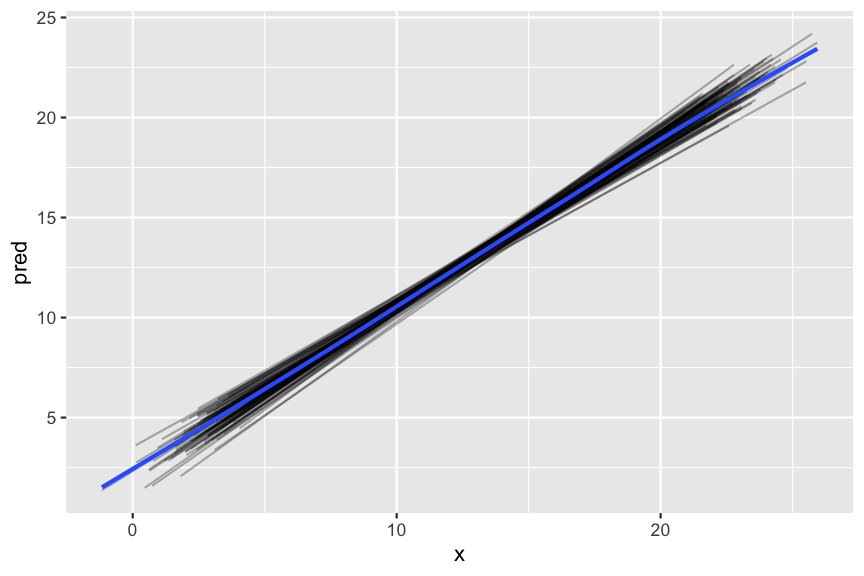Example DATRAS data

• Restructure the dataset to create a nested data frame grouped by species.
• Apply purrr's mapping function to model the species CPUE as a function of latitude.
• Save the models and summary statistics in the same nested dataframe.
• Generate ggplots with the predicted CPUE ~ lat per species and save these in a list-column.
• Identify the species where the CPUE is best explained by latitude and look at the prediction plot.

Overview of functions you learned today

purrr functions:
map(), map_lgl(), map_int(), map_dbl(), map_chr()
set_names()
map2(), map2_lgl(), map2_int(), map2_dbl(), map2_chr()
pmap(), invoke_map(), invoke_map_dbl()
invoke(), lmap(), imap(), walk(), walk2(), pwalk()

tidyr functions:
nest(), unnest()

How do you feel now.....?

Totally bored?Then apply more of the map functions to your second case study!

Totally content?

Then go grab a coffee, lean back and enjoy the rest of the day...!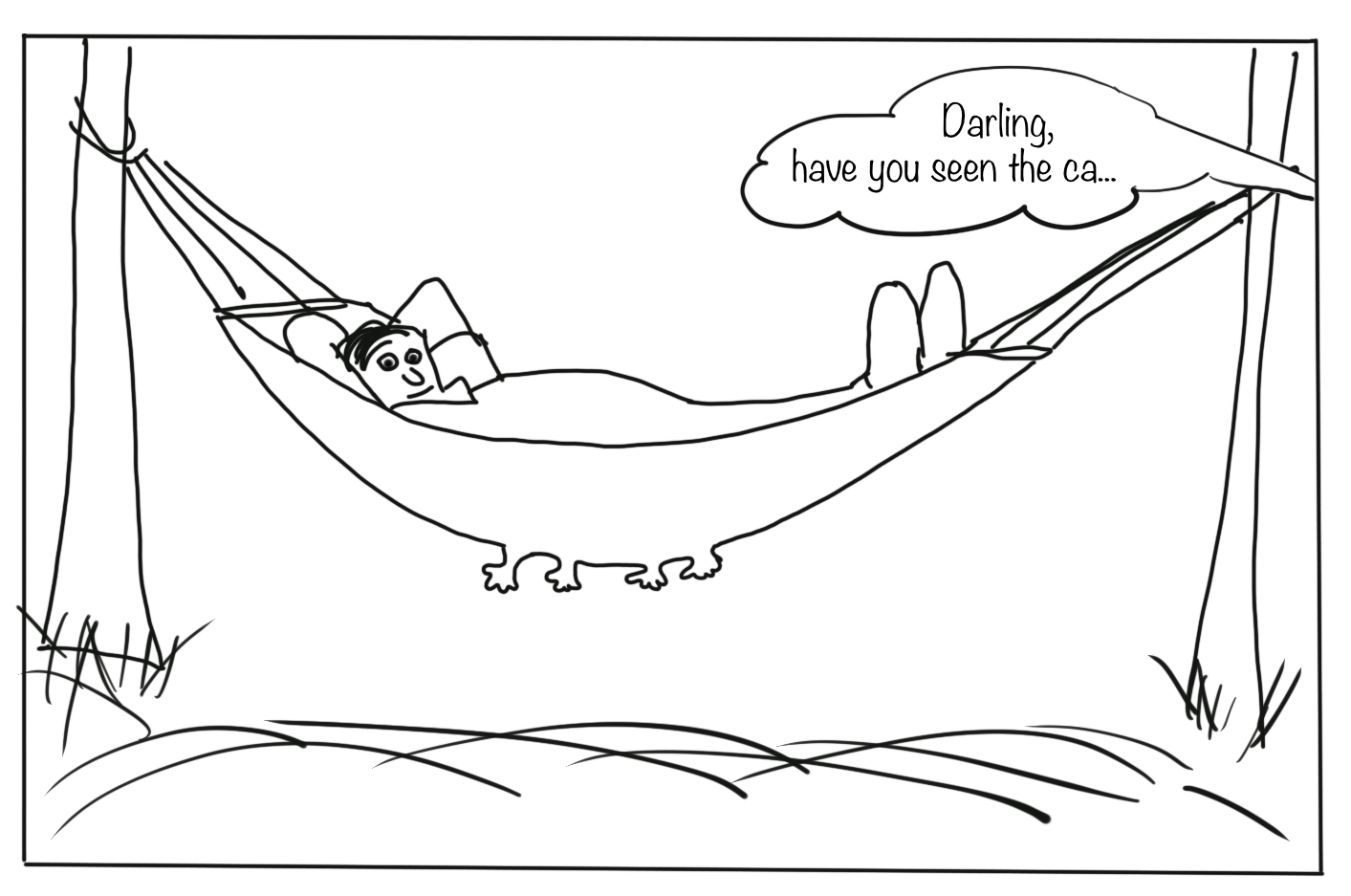Thank You